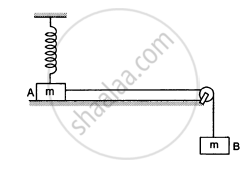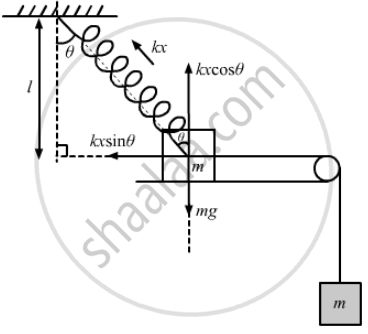Advertisement Remove all ads

# Figure Following Shows Two Blocks a and B, Each of Mass of 320 G Connected by a Light String Passing Over a Smooth Light Pulley. the Horizontal Surface on Which the Block Acan Slide is Smooth. - Physics

Numerical

In the following figure shows two blocks A and B, each of mass of 320 g connected by a light string passing over a smooth light pulley. The horizontal surface on which the block Acan slide is smooth. Block A is attached to a spring of spring constant 40 N/m whose other end is fixed to a support 40 cm above the horizontal surface. Initially, the spring is vertical and unstretched when the system is released to move. Find the velocity of the block A at the instant it breaks off the surface below it. Take g = 10 m/s2.Advertisement Remove all ads

#### Solution

$\text{ Given }:$
$\text{ Mass of each block, m = 320 g = 0 . 32 kg }$
$\text{ Spring constant, k = 40 N/m }$
$\text{ h = 40 cm = 0 . 4 m and g = 10 m/ s }^2$From the free-body diagram,

$\text{ kx } \cos \theta = \text{ mg }$

As, when the block breaks of the surface below it (i.e. gets dettached from the surface) then  R =0.

$\Rightarrow \cos \theta = \frac{\text{ mg }}{\text{ kx }}$
$\Rightarrow \frac{0 . 4}{0 . 4 + \text{ x }} = \frac{3 . 2}{40 \text{ x }}$
$\Rightarrow 16\text{x = 3 . 2 x }+ 1 . 28$
$\Rightarrow \text{ x = 0 . 1 m }$
$\text{ So, s = AB }= \sqrt{\left(\text{ h + x}^2 \right) - \text{h}^2}$
$= \sqrt{\left( 0 . 5 \right)^2 - \left( 0 . 4 \right)^2} = 0 . 3 \text{m}$

Let the velocity of body B be $\nu$ .

Change in K.E. = Work done (for the system)

$\left( \frac{1}{2} \text{mu}^2 + \frac{1}{2}\text{m} \nu^2 \right) = - \frac{1}{2}\text{kx}^2 + \text{mgs}$
$\Rightarrow \left( 0 . 32 \right) \times \nu^2$
$= - \left( \frac{1}{2} \right) \times 40 \times \left( 1 . 0 \right)^2 + \left( 0 . 32 \right) \times 10 \times \left( 0 . 3 \right)$
$\Rightarrow \nu = 1 . 5 \text{m/s}$

From the figure,

$∆ \text{ l = h }\left( \sec \theta - 1 \right) . . . \left( \text{ i } \right)$
From the principle of  conservation of energy,
$\text{mgs} = 2\left[ \frac{1}{2}\text{m}\nu^2 \right] + \frac{1}{2} \text{K ∆ l}^2$
$\text{ mgh } \tan \theta = \text{m} \nu^2 + \frac{1}{2}\text{kh}^2 \left( \sec \theta - 1 \right)^2 . . . (\text{ii})$
When the motion of the block breaks of the surface below it (i.e gets dettached from the surface on which it was initially placed) then
$\text{ mg = kh } \left( \sec \theta - 1 \right) \cos \theta$
$\Rightarrow 1 - \cos \theta = \frac{\text{mg}}{\text{kh}}$
$\Rightarrow \cos \theta = 1 - \frac{\text{mg}}{\text{kh}}$
$\text{or} \cos \theta = \frac{\text{ kh - mg }}{\text{ kh } }$
$= \frac{40 \times 0 . 4 - 0 . 32 \times 10}{40 \times 0 . 4}$
$= 0 . 8$
Putting the value of θ in equation (ii), we get:

$0 . 32 \times 10 \times 0 . 4 \times 0 . 75$

$= 0 . 32 \nu^2 + \frac{1}{2}40 \times \left( 0 . 4 \right)^2 \left( 1 . 25 - 1 \right)^2$

$\Rightarrow 0 . 96 = 0 . 32 \nu^2 + 0 . 2$

$\Rightarrow 0 . 32 \nu^2 = 0 . 72$

$\Rightarrow \nu = 1 . 5 \text{ m/s }$

Is there an error in this question or solution?
Advertisement Remove all ads

#### APPEARS IN

HC Verma Class 11, 12 Concepts of Physics 1
Chapter 8 Work and Energy
Q 50 | Page 135
Advertisement Remove all ads
Advertisement Remove all ads
Share
Notifications

View all notifications

Forgot password?